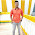### The Ayrton Shunt or Universal Shunt

we have seen that in multirange ammeter, a make before break switch is must. The Ayrton shunt or universal shunt eliminates the possibility of having a meter without a shunt. The meter with Ayrton shunt is shown in the Fig. 1.

The selector switch S, selects the appropriate shunt required to change the range of the meter. When the position of the switch is '1' then the resistance R1 is in parallel with the series combination of R2 R3 and Rm. Hence current through the shunt is more than the current through the meter, thus protecting the basic meter. When the switch is in the position '2', then the series resistance of R1 and R2 is in parallel with the series combination of R3 and Rm. The current through the meter is more than through the shunt in this position. In the position '3', the resistances R1 R2 and R are in series and acts as the shunt. In this position, the maximum current flows through the meter. This increases the sensitivity of the meter.
The voltage drop across the two parallel branches is always equal.
Thus, Ish Rsh Im  Rm
But in position 1, R1 is in parallel with R2 R3 Rm

where I1 is the first range required.
In position 2, R1 R2 is in parallel with R3 Rm .

where I2 is the second range required.
In position 3, R1 R2 R3 is in parallel with Rm .

where I3 is the third range required.
The current range I3 is the minimum while I1 is maximum range possible. Solving the equations (1), (2) and (3) the required Ayrton shunt can be designed.

1.1 Precautions to be Taken While Using an Ammeter
The following precautions must be taken while using an ammeter.
1) As the ammeter resistance is very low, it should never be connected across any source of e.m.f. Always connect an ammeter in series with the load.
2) The polarities must be observed correctly. The opposite polarities deflect the pointer in opposite direction against the mechanical stop ans this may damage the pointer.
3) While using multirange ammeter, first us e the highest current range and then decrease the current range until sufficient deflection is obtained. So to increase the accuracy, finally select the range which will give the reading near full scale deflection.

1.2.